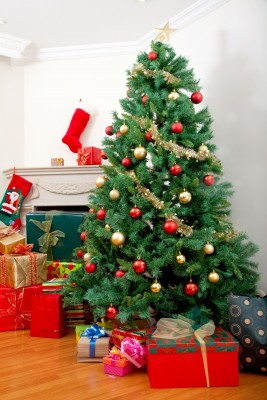A formula has been derived for creating the perfectly decorated Christmas tree regardless of size. The formula has been named 'Treegonometry'.

If you want to put the theory to the test, here's what you need to know...

1. The angle/star on the top of the tree needs to be one tenth of the height of the tree itself.

2. For the ideal number of lights take the height of the tree in centimeters and multiply by 3.14 (Pi).

3. To know how much tinsel to get simply multiply 13 by 3.14 (Pi), divide by 8, then multiply by the height of the tree in centimeters

4. To find out how many baubles you'll need take the square root of 17 (which is 4.12), divide it by 20 and multiply it by the height of the tree in centimeters.

So, for a 6ft tree (183cm), you will need 575 lights, a star or an angel which is 18cm tall, 934cm of tinsel and 37 baubles.It was Debenhams who set the challenge to the University of Sheffield, whose Maths Society came up with the formula. So now we can all have a perfectly decorated Christmas tree which is neither too green nor too glitzy.

Debenhams Christmas decorations buyer Sarah Theobold commented:
The formula is so versatile it will work for a tree large enough for the Royal Family at Balmoral, but also on trees small enough for the most modest of homes.
Customers are often making the error of buying too large or small an angel; however this simple formula means you’ll have the tree to star ratio correct.

If you' like to put the theory to the test we would love to see photos of the results!

Topics: eFlorist News